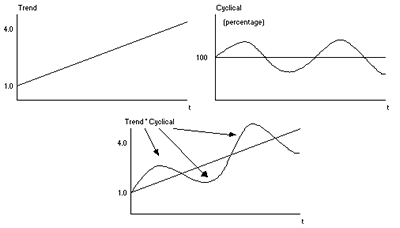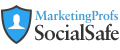# Real-World Education for Modern Marketers

## Join Over 600,000 Marketing Professionals

Text:  A A
 Forecasting Guide Overview Categories Selecting the Approach Combining Approaches Judgmental Models Delphi Method Curve Fitting Analogous Data Time Series Models Moving Average Exponential Smoothing Decomposition Models Box-Jenkins Models Regression Leading Indicator Input-Output Models
TECHNIQUE #7: Decomposition Models

BASIC IDEA: This forecasting approach is based on the idea that a forecast can be improved if the underlying factors of a data pattern can be identified and forecasted separately. Breaking down the data into its component parts is called decomposition. The decomposition model assumes that sales are affected by four factors: the general trend in the data, general economic cycles, seasonality, and irregular or random occurrences. The forecast is made by considering each of these components separately and then combining them together.

PROCEDURE: To make things easy, let's assume that seasonality is not a major consideration in the forecast (for example, you are making annual forecasts) and irregular occurrences can be assumed to smooth out over the forecast horizon. Then, decomposition begins by assuming that the data can be represented by a trend and cyclic component which are combined in a multiplicative fashion:

Sales(t) = Trend(t) * Cyclical(t)

Pictorially, the combined trend*cyclical pattern is being decomposed into its constituent elements, as shown below. The cyclic portion is simply the percentage of the trend that is due to cyclic elements. Here it is represented by a simple sine wave, which is a loose representation of cyclic behavior. The difficult part is estimating the size of the peaks and troughs as well as the exact timing.Given this as background, the procedure is as follows:

• Develop a trend line through the data to get a value for the trend, perhaps by using a simple trend analysis done by freehand or using regression analysis (described in Technique #9).

Determine the cyclical component of the data. This can be done by dividing the shipments at every point by the value of the trend at that point (i.e. cyclet=shipmentt/trendt) The references provide several other methods for doing this.

• To make a forecast, recombine the components: Provide separate forecasts for sales due to the trend and the cyclical component for next period and multiply as in the above formula.

example: Sales data between 1976 and 1989 are graphed below. The straight line (which was calculated using regression analysis) represents the trend line for this series of data points. Again, a trend line could also be developed by freehand. Note how the data cycles above and below the trend line.

For each point, the actual data was then divided by the value of the trend line at that point to yield a value of the cycle "ratio" . This is graphed below:

To make a forecast for 1990, we need a forecast for the trend component, which can be estimated from the trend line. In this case, the regression equation provides the estimate for 1990, which is 14051. The cyclic portion is more difficult, however given the nature of the historical cyclic behavior, we might expect the cycle "ratio" to turn down, perhaps to a value of 1.0. Then our sales estimate for 1990 would be (plugging the trend and cycle estimates into the above equation for sales): 14051*1.00 = 14051.

• Incorporation of seasonality is relatively straightforward, and necessary for quarterly sales forecasts that may be subject to seasonality. See the references for how to do this.

• Additive models have been used also, but the multiplicative model shown above is more popular.

• As with all time series models, explanatory factors not included.

Search over 4,000 MarketingProfs Resources!

## Subscribe Today

IT'S FREE! Become a member to get the tools and knowledge you need to market smarter.

## Stay connected!

Don't miss a bit of MarketingProfs ... join the 600,000 marketers following us.

## Popular Marketing Topics

### Get to the Po!nt NewslettersMarketingProfs uses single